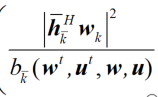# How to solve {positive constant}/ {complex affine}

Hello everyone, I encountered some difficulties while solving this problem. I hope to get your help. Thank you.
My Formula

My Code

``````cvx_begin
variable w(M,K) complex
for k=1:K
w_temp5=0;
for i=k+1:K
w_temp5=w_temp5+pow_abs((u_t'*H(:,:,i)+h_Bk(:,i)')*w_t(:,i),2);
end
for p=k+1:K
w_temp7=pow_abs((u_t'*H(:,:,p)+h_Bk(:,p)')*w_t(:,k),2);
w_temp6=0;
for j=k+1:K
w_temp6=w_temp6+2*real((h_Bk(:,j)' +u_t'*diag(h_rk(:,j)')*G)*w_t(:,j)*w(:,j)'*(h_Bk(:,j)'+u'*diag(h_rk(:,j)')*G)')...
-((h_Bk(:,j)' +u_t'*diag(h_rk(:,j)')*G)*w_t(:,j)*w_t(:,j)'*(h_Bk(:,j)'+u_t'*diag(h_rk(:,j)')*G)');
end
RR(k,p)=log(1+(w_temp7)/(w_temp5+noise))/log(2)+...
((w_temp7)/(w_temp6+noise)...
-(w_temp7)/(w_temp5+noise))/(log(2)+(w_temp7*log(2))/(w_temp5+noise));
end
end
``````

I don’t know what is done with this stuff, once defined. Nor do I understand the symbols and conventions used. Are you applying `real(....)` everywhere it is needed? You can’t divide by a variable or CVX expression in CVX, but you can use `inv_pos` if you follow its rules (its argument must be concave, which includes affine as a special case of concave, and only makes sense if the expression to which it is applied is known to be positive).

Have you proven the problem is convex? That is the first step. Don’t answer that you have proven it is convex until you carefully read

Thank you very much, mark, but I found that I made a mistake.``````w_t  is an initial value
variable w(M,K) complex
w_temp8=pow_abs(h_k(:,k+1)'*w(:,k),2)
w_temp6=2*real(h_k(:,k+1)' *w_t(:,k+1)*w(:,k+1)'*h_k(:,k+1))...
-h_k(:,k+1)' *w_t(:,j)*w_t(:,k+1)'*h_k(:,k+1);
r(k)=w_temp8/w_temp6;

``````

I use the function quad_ over_ Lin, an error occurred

`quad_over_lin(x,y)` replaces `x'*x/y`. Both `x` and `y` must be affine. The numerator of the modeled expression is quadratic (`x'*x`), but the first argument provided to `quad_over_lin` is affine, not quadratic.

I am omitting al the subscripts, which i will let you deal with appropriately
`quad_over_lin(h'*w,b)`
I don’t understand what `b` really is. In order for this formulation to work, `b` needs to be affine int the optimization (CVX) variables, which means when all CVX expressions are written in terms of CVX optimization variables.

Thank you very much, mark. The problem has been solved